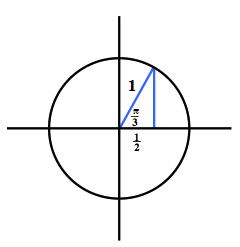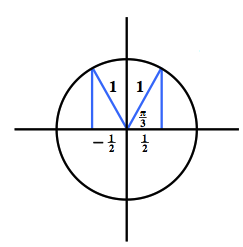### Home > CCA2 > Chapter Ch10 > Lesson 10.1.4 > Problem10-69

10-69.

Use reference angles, the symmetry of a circle, and the knowledge that cos$(\frac { \pi } { 3 })=\frac { 1 } { 2 }$ to write three other true statements using cosine and angles that are multiples of $\frac { \pi } { 3 }$.

Draw a unit circle diagram for the given angle. Unit circle, with right triangle in first quadrant, labeled as follows: hypotenuse, 1, horizontal leg, 1 half, angle opposite vertical leg, pi thirds.

Reflect the triangle in each of the $4$ quadrants. Quadrant $2$ is shown for you. Added to the unit circle, a right triangle in second quadrant, labeled as follows: hypotenuse, 1, horizontal leg, negative 1 half.

$\text{cos}\left( \frac{2\pi}{3} \right)=-\frac{1}{2}$